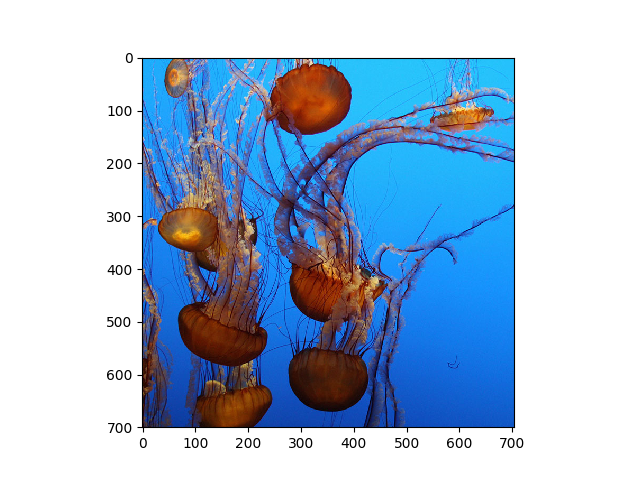This example is a bit contrived, but it demonstrates the basic features of a Trieste Collection in a simple way. We will load a color image, break it into three channels (R, G, B), and store each channel in a 2-D Trieste Array. The three Arrays will then be stored in a Collection, which will be saved to disk and then read from disk. Finally, the contents of the file will be examined.

In :
%pylab notebook

Populating the interactive namespace from numpy and matplotlib

In :
import trieste as tr


In :
image = plt.imread("IMG_2054.jpg")


This is what the image looks like:

In :
plt.imshow(image)Out:
<matplotlib.image.AxesImage at 0x7f036a671630>
In :
plt.close()


The image object is a 3-dimensional NumPy array. We can decompose it into red, green and blue channels:

In :
red = image[:,:,0]

green = image[:,:,1]

blue = image[:,:,2]


Now, we can store each channel in a Trieste Array:

In :
R = tr.Array(red)

G = tr.Array(green)

B = tr.Array(blue)


These three 2-dimensional Array objects can be stored together in a Collection. Let's add some metadata---a caption:

In :
meta = {'caption': 'This is a caption for the photo taken in 2010 at the Monterey Bay Aquarium.'}

In :
jelly = tr.Collection([R,G,B], name='jellyfish', metadata=meta)


You can add a documentation string / README page to the collection to explain what it is:

In :
read_me = """
This is potentially a long document describing the content of this Collection.
"""

In :
jelly.set_readme(read_me)


Save the collection as a file:

In :
jelly.save_as('jellies')


Now, we can load the file:

In :
J = tr.load('jellies.npz')

In :
J.name

Out:
'jellyfish'

Since the file only contains one top-level object (a Collection), the load() function creates a Collection object, rather than a File object:

In :
J

Out:
<jellyfish: a Collection of 3 2-D Arrays>
In :
type(J)

Out:
trieste.core.Collection
In :
print(J.readme)

This is potentially a long document describing the content of this Collection.



The table of contents for the Collection:

In :
J.toc

Out:
['red', 'green', 'blue']

A Collection can be indexed using the name of an object that it contains, as in:

In :
J['red']

Out:
<red: a Trieste 2-D Array>
In :
plt.imshow(J['blue'].data, cmap='hot')